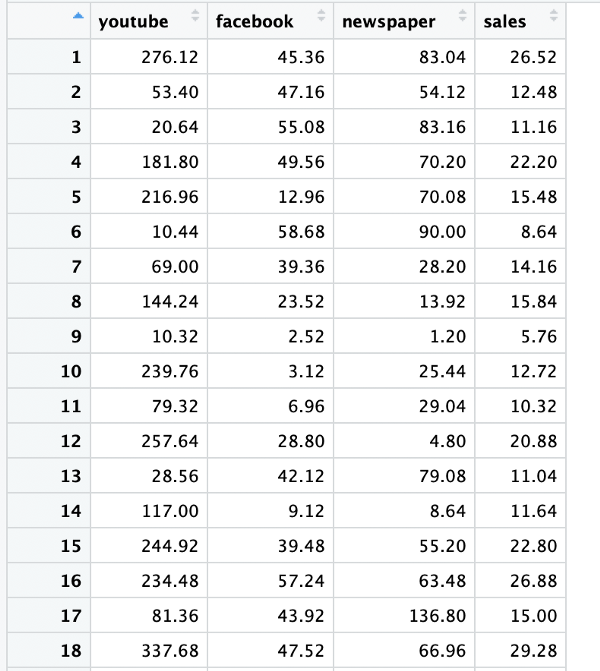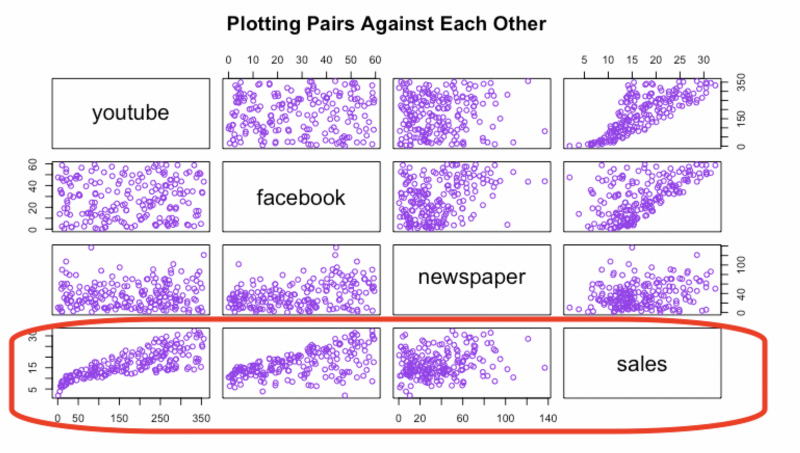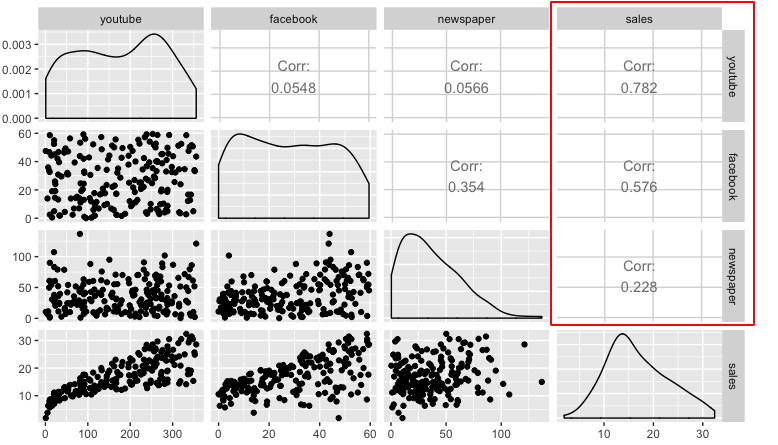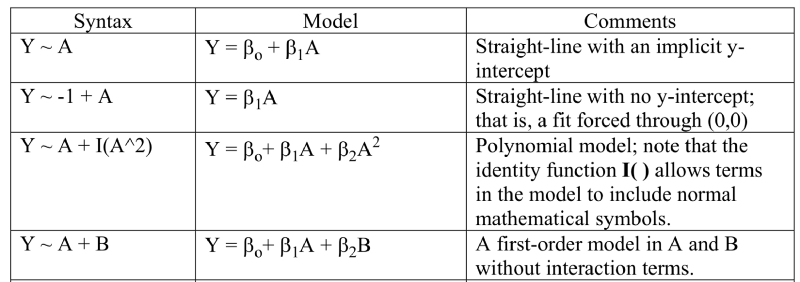### A beginner’s tutorial to perform linear regression and plotting in R

Linear regression is the first step most beginners take when starting out in machine learning.

The article above explains the theory behind linear regression beautifully. Today, however, we are going to see how to actually perform linear regression in code. The complete code is given at the bottom of the article.

The language of choice for this article is R given its beautiful plotting packages but python would be equally convenient.

To write R code we use RStudio. The beautifully organized RStudio lets us browse some preloaded datasets in R. We search for the datarium package

To install it we use the R command to install any package

``install.packages("datarium")``

Looking into the details of the package we see the following datasets. The dataset of interest for us is called marketing. It is a dataset containing the impact of three advertising medias (youtube, facebook and newspaper) on sales. The first three columns are the advertising budget in thousands of dollars along with the fourth column as sales. The advertising experiment has been repeated 200 times. Hence, it has 200 rows.Dataset Description — showing the first 18 rows

We will structure the code as the following 4sections

1. Loading and understanding the dataset
2. Preparing the data
3. Building the model
4. Model accuracy analysis

### 1. Loading and understanding the data

``````#The Libraries required
install.packages("datarium")#Packages need to be installed only once
install.packages("caTools")
install.packages("ggplot2")
install.packages("GGally")

#Loading The Data
data("marketing", package = "datarium")
data_size = dim(marketing)``````

Since there are 200 rows and 4 columns in the data corresponding to youtube, Facebook, newspaper and sales the data size comes out to be 200 x 4.

### Understanding the data

Once it is loaded it makes sense to look at a few properties of the data. The very first thing people do is to look at a few samples of the dataset. A command called head is used.

A command called summary gives you the basic statistics of your dataset like mean, median, 1st quartile, 2nd quartile etc.

Since this is a linear regression experiment which involves looking at how sales vary with the advertising budget, it makes sense to see the trends of all the 3 advertising channels.Plotting the data pairwise

The last row (highlighted) of plots is the most useful. It indicates how various advertising channel budgets impact the sales. We can clearly see that youtube and facebook sales (1st two plots in the highlited row) increase linearly with increase in the advertising budget. The newspaper (3rd plot) sales however show no particular trend.

R has another useful plotting library called ggplot. Using ggplot we can draw the same information in a slightly different manner.Correlation between each media and corresponding sales numbers

The diagonal consists of the densities of the three variables and the upper panels consist of the correlation coefficients between the variables. Looking at the highlighted correlation numbers we can see that youtube (0.78) and facebook (0.58) have a much higher correlation to sales than newspapers (0.23).

The code for the above plots and summaries is as follows

``````#Understanding the data
head(marketing)
summary(marketing)

#Pairwise plotting technique 1
plot(marketing, col="purple", main="Plotting Pairs Against Each Other")

#Pairwise plotting technique 2
ggpairs(marketing)``````

### 2. Preparing the data

``````#Global Variable
splitRatio = 0.75

#Splitting The Data
set.seed(101)# Set Seed so that same sample can be reproduced in future also

#Now Selecting 75% of data as sample from total 'n' rows of the data
sample = sample.split(marketing\$youtube, SplitRatio = splitRatio)
train = subset(marketing, sample == TRUE)
test = subset(marketing, sample == FALSE)
train_size = dim(train)
test_size = dim(test)``````

To prepare the data we split the data into training and testing sets. If we choose the splitting ratio as 50 percent then 100 samples go into training set and 100 into the test set. For our case we choose the splitting ratio as 75 percent. So after the split we have

• Training data = 150 rows * 4 columns
• Test data = 50 rows * 4 columns

The code for splitting the data uses a library called caTools which randomly takes fixed number of samples from the original dataset and splits it.

The environment section of RStudio shows us the variable sizes

### 3. Creating the model

``````#creating the model
Model <- lm(sales ~ youtube + facebook + newspaper, data = marketing)
summary(Model)``````

A case of multiple linear regression we have ‘n’ variables that combine linearly to provide us with our output. Its equation looks like the following. Betas (ß) are the coefficients that control the effect of any variable (x) has on the output (y)

If n equals 1 then this becomes simple linear regression that is explored in this article. The equation for that reduces to the form y=mx+c

In the marketing dataset however, we have three different variables — namely, youtube, Facebook and newspaper. Hence its equation would look something like this

For our use case this would become

The goal for us would be to find the betas (ß) and determine how accurately they predict sales numbers. Now that we know the equation how do we translate that to code. The key to that lies in understanding how we define linear models in R. The table below shows how to translate a few different model types into code.Source

This would mean that the code for our model would look like this

``sales ~ youtube + facebook + newspaper``

### Interpreting the model

The summary function in R returns model properties. If we take a look at the model we will see some peculiar properties.

The first step in interpreting the multiple regression analysis is to examine the F-statistic and the associated p-value, at the bottom of model summary.

In our example, it can be seen that p-value of the F-statistic is < 2.2e-16. This small number means that, at least, one of the predictor variables (youtube, FB or newspaper) is significantly related to the outcome variable.

It can be seen that from the estimates column that, changes in the youtube and facebook advertising budgets are significantly associated to changes in sales while changes in the newspaper budget is not. We saw this also when we plotted our variables pairwise. As an example, spending an additional 1000 dollars on facebook advertising leads to an increase in sales by approximately 0.1885*1000 = 189 sale units, on average. The youtube coefficient suggests that for every 1000 dollars increase in budget we can get an increase of 0.045*1000 = 45 sales units, on average.

The same value for newspaper is small and is not significant in the multiple regression model. Thus we can remove newspaper completely from our analysis.

This would mean that our final model equation would look like

sales = 3.5 + 0.045*youtube + 0.187*facebook

### 4. Model Accuracy Analysis

Now that we finally have our model it is time to test how good it actually is.

``````#Predicting
pred <- predict(Model, test)
numx <- data_size*(1 - splitRatio)
x_axis <- seq(numx)
df <- data.frame(x_axis, pred,test\$sales)

#Plotting the predicted values against the actual values
g <- ggplot(df, aes(x=x_axis))
g <- g + geom_line(aes(y=pred, colour="Predicted"))
g <- g + geom_point(aes(x=x_axis, y=pred, colour="Predicted"))
g <- g + geom_line(aes(y=test\$sales, colour="Actual"))
g <- g + geom_point(aes(x=x_axis, y=test\$sales, colour="Actual"))
g <- g + scale_colour_manual("", values = c(Predicted="red", Actual="blue"))
g``````

One way to look at how good the model is to use our test set that we had separated out before and make the model predict sales values for those data points. Since we have the actual sales numbers for the same points we can compare them to qualitative see our model’s performance. We can see in the below graph how close our red points (predictions) to the blue ones (actual).

Even though we can see the closeness this is still a qualitative way to evaluate the model. To put a number to model’s accuracy we use the following statistical terms. Note that terms like precision, recall, sensitivity and specificity are used more commonly for neural networks and SVMs as described in this article.

Regression model evaluation metrics

The MSE, MAE, RMSE, and R-Squared metrics are mainly used to evaluate the prediction error rates and model performance in regression analysis.

• MAE (Mean absolute error) represents the difference between the original and predicted values. We get this number by averaging the absolute difference over the data set.
• MSE (Mean Squared Error) represents the difference between the original and predicted values extracted by averaging the squared difference over the data set.
• RMSE (Root Mean Squared Error) is the square root of the the arithmetic mean of the squares of difference over the set.
• R-squared (Coefficient of determination) represents the coefficient of how well the values fit compared to the original values. The value from 0 to 1 interpreted as percentages. The higher the value is, the better the model is.
``````#Evaluation
original = test\$sales
predicted = pred
d = original-predicted
mse = mean((d)^2)
mae = mean(abs(d))
rmse = sqrt(mse)
R2 = 1-(sum((d)^2)/sum((original-mean(original))^2))

cat(" MAE:", mae, "\n", "MSE:", mse, "\n",
"RMSE:", rmse, "\n", "R-squared:", R2)``````

For our model R-Squared error comes out to be 0.88 which is a pretty good number but not probably the best in the world.

Linear regression is generally a great way to get a hang of the field of machine learning and statistics. It is a quick and easy way to understand a dataset. R as a language is very versatile when it comes to data analysis and visualization. Here’s a Github link for anyone who wants to go through the complete code.

X8 aims to organize and build a community for AI that not only is open source but also looks at the ethical and political aspects of it. More such simplified AI concepts will follow. If you liked this or have some feedback or follow-up questions please comment below.

Thanks for Reading!